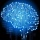Home Communities
IT Knowledge
Inspiration
Languages
EN

# Java Base64 encode and decode example

1 points
Created by:Root-ssh
125480

Hello everyone 👋.

Today I would like to show you how to use java Base64 class to encode and decode String in super simple and short way.

Here we go.

## 1. Java 8 - Base64 Util

Note: Base64 util was added in Java 8.

``````import java.util.Base64;

public class Example {

public static void main(String[] args) {

String text = "test123";

// encode
String encodedString = Base64.getEncoder().encodeToString(text.getBytes());
System.out.println(encodedString); // dGVzdDEyMw==

// decode
String decodedString = new String(Base64.getDecoder().decode(encodedString));
System.out.println(decodedString); // test123
}
}``````

Output:

``````dGVzdDEyMw==
test123``````

Some explanation to coders that here about Base64 for the first time, or to refresh our knowledge.

In above example we use text as out input. First we encode it and then we decode it.

As we can see our text is "test123" and when we encode this string with base64 it is equal to "dGVzdDEyMw==".

Everytime when we decode this string "dGVzdDEyMw==" with base64 decoder we will get our initial text "test123". It is programming language independed, we can also use online base64 encoder / decoder to see if it works as expected.

What is Base64 in general? From wikipedia we can see:

Base64 is a group of binary-to-text encoding schemes that represent binary data in an ASCII string format by translating it into a radix-64 representation.

https://en.wikipedia.org/wiki/Base64

## 2. Base64 - encode / decode - byte array

In this example we take String as input, encode it to byte array and decode from encoded byte[] to decoded byte[].

``````import java.util.Base64;

public class Example2 {

public static void main(String[] args) {

String text = "test123";

// encode to byte array
byte[] encode = Base64.getEncoder().encode(text.getBytes());

String encodedString = new String(encode);
System.out.println(encodedString); // dGVzdDEyMw==

// decode to byte array
byte[] decode = Base64.getDecoder().decode(encode);

String decodedString = new String(decode);
System.out.println(decodedString); // test123
}
}``````

Output:

``````dGVzdDEyMw==
test123``````